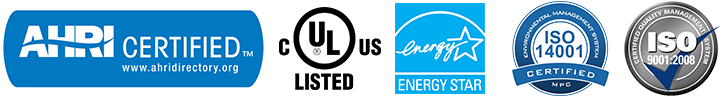# Pioneer® Quint Zone Outdoor Section 230V 21.5 SEER Multi Split Air Conditioner & Heat Pump

Model Number: YN050GMFI22M5D

### Limited Time Offer!

1. Free Shipping within the Continental US
2. Pre-Charged for line lengths up to 25 ft long per circuit
3. Wholesale Price Directly from Factory

THIS IS AN OUTDOOR SECTION ONLY, YOU NEED TO ALSO PURCHASE A COMPATIBLE INDOOR SECTION IN ORDER TO HAVE A COMPLETE WORKING SYSTEM.

• \$3,098.00
• Save \$992

### Summary

• OUTDOOR SECTION ONLY for Quint (5) Zone
• Ultra High Efficiency DC Inverter++ version
• Cooling capacity: 48,000 BTU/H Nominal (Range: 9,650 MIN ~ 53,500 MAX) with 21.5 SEER efficiency
• Heating capacity: 48,000 BTU/H Nominal (Range: 6,850 MIN ~ 53,980 MAX) with 10.4 HSPF efficiency
• Voltage: 208~230 V, 60 Hertz, 1 Ph
• Factory charged for up to 25’ line set per circuit
• Whisper Quiet Operation

### Refrigerant

R410A Pre-Charged Outdoor Unit, accommodating refrigerant line lengths up to 25 ft. per circuit.

### Certifications### Performance Data

• Power Supply: 208~230V, 60Hz, 1Ph
• Communication Protocol: Current Loop
• Min-Max Load Attached: 36,000~64,000 BTU/h
• Cooling Rated Capacity: 48,000 BTU/h
• Cooling Capacity Range: 9,650~53,500 BTU/h
• Cooling SEER (Ductless): 21.5 BTU/w
• Heating Rated Capacity: 48,000 BTU/h
• Heating Capacity Range: 6,850~53,980 BTU/h
• Heating HSPF (Ductless): 10.4 BTU/w
• Refrigerant Type: R410a
• Suitable Area: 1,600~2,000 Sq.F
• Sound Level: 64.0 dB(A)
• Net Dimensions (WDH): 37.2 x 16.4 x 52.5 Inches
• Carton Dimensions (WDH): 43.2 x 19.5 x 58.3 Inches
•  Net / Gross Weight: 224 / 255 Lbs

### Quint Zone Combinations

Rating Two Units Three units Four units Five units
Wall: 9 + 9 + 9 + 9 + 9 18 + 18 9 + 9 + 18 9 + 9 + 9 + 9 9 + 9 + 9 + 9 + 9
Ducted: 9 + 9 + 9 + 9 + 9 18 + 24 9 + 9 + 24 9 + 9 + 9 + 12 9 + 9 + 9 + 9 + 12
24 + 24 9 + 12 + 12 9 + 9 + 9 + 18 9 + 9 + 9 + 9 + 18
9 + 12 + 18 9 + 9 + 9 + 24 9 + 9 + 9 + 9 + 24
9 + 12 + 24 9 + 9 + 12 + 12 9 + 9 + 9 + 12 + 12
9 + 18 + 18 9 + 9 + 12 + 18 9 + 9 + 9 + 12 + 18
9 + 18 + 24 9 + 9 + 12 + 24 9 + 9 + 9 + 18 + 18
9 + 24 + 24 9 + 9 + 18 + 18 9 + 9 + 12 + 12 + 12
12 + 12 + 12 9 + 9 + 18 + 24 9 + 9 + 12 + 12 + 18
12 + 12 + 18 9 + 12 + 12 + 12 9 + 12 + 12 + 12 + 12
12 + 12 + 24 9 + 12 + 12 + 18 9 + 12 + 12 + 12 + 18
12 + 18 + 18 9 + 12 + 12 + 24 12 + 12 + 12 + 12 + 12
12 + 18 + 24 9 + 12 + 18 + 18
12 + 24 + 24 9 + 18 + 18 + 18
18 + 18 + 18 12 + 12 + 12 + 12
18 + 18 + 24 12 + 12 + 12 + 18
12 + 12 + 12 + 24
12 + 12 + 18 + 18

### 24,000 BTU Compatible Units

32,000 / 48,000 BTU/h.

### Limitations

Do not exceed two pieces of 24,000 BTU/h Indoor Unit

### Notes

Every indoor unit attached will operate at a random, self regulated capacity, based on the actual demand it measures from the zone it is serving (Between 30% to 100% of its rated capacity or OFF). Outdoor unit will also self regulate its output capacity, based on the total demand it reads from all of the simultaneously running indoor units at any given moment.

With multi split systems, the total demand from the outdoor unit, shall seldomly exceed 67% of the total available capacity of the combined indoor unit group due to load fluctuations. Although very seldom, if and when the total demand from the combined indoor group exceeds the rated capacity of the outdoor unit, the capacity of the each indoor unit will be annotated accordingly.

For high demand heating applications, max loading limits may need to be reduced by 10-15 % to avoid under-performance risks at some extreme conditions.

• How many AMPS does this unit require?

This system takes a 40A double-pole breaker.

• What is the minimum outdoor temp for heating and cooling?

This unit can COOL down to +5°F outside temperatures and HEAT down to -13°F outside temperatures.

## Product Reviews from Verified Customers

Customer Reviews View on Amazon

out of 5 stars

5 star

4 star

3 star

2 star

1 star